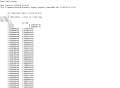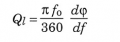# resonance frequency and quality factor of a harmonic oscillation

#### Papabravo

Joined Feb 24, 2006
20,618
The resonant frequency is calculated from the component values used to construct the harmonic oscillator. The differential equation is the same one as the mechanical spring-mass system.
The quality factor is computed from the resonant frequency and the bandwidth of the magnitude response.

Joined May 11, 2018
158
The resonant frequency is calculated from the component values used to construct the harmonic oscillator. The differential equation is the same one as the mechanical spring-mass system.
The quality factor is computed from the resonant frequency and the bandwidth of the magnitude response.
so
The resonant frequency is calculated from the component values used to construct the harmonic oscillator. The differential equation is the same one as the mechanical spring-mass system.
The quality factor is computed from the resonant frequency and the bandwidth of the magnitude response.
Hello can you elaborate a little bit what you mean by the components used to construct the harmonic oscillatorthes are the values that i used to draw the frequency gaussian

#### neonstrobe

Joined May 15, 2009
189
You need to look at the capacitor and inductor values.L and C. The frequency response could be made up from a number of different combinations.

#### Papabravo

Joined Feb 24, 2006
20,618
so

Hello can you elaborate a little bit what you mean by the components used to construct the harmonic oscillator
View attachment 227475
thes are the values that i used to draw the frequency gaussian
I have no idea what a "frequency gaussian" is. It sounds like a term you just made up. There are no units on your numbers. who can say what they represent.
Electronic Oscillators are made from real components, like resistors with units of Ohms, capacitors with units of Farads, and inductors with units of Henries, and amplifiers . Mechanical oscillators are made from springs and masses. These things all have units of measurement.

#### MisterBill2

Joined Jan 23, 2018
16,626
And what is a "harmonic oscillator"??? The frequency is mostly determined by the inductance and capacitance values, but phase shift in the gain portion of the circuit seems to have an effect as well.
But that big table of numbers mentions a PLL which is different, with some of them being voltage controlled osillators instead of LC types.

#### sparky 1

Joined Nov 3, 2018
742
I am not an expert I understand only some of the basics used in (spm) statistical parametric mapping.
spm image preprocessing uses smoothing like digital filtering of photos.

In one period initiating certain (NMR frequencies) a DAC catches artifacts because the field's excitation on subparticles.
Matlab has a collection of algorithms that analyze the gradient's echos, these effects are found by statistical study.
A single precession might look like the animation below. When many of these begin to spin down they show up in the data.

To visualize going from time domain to frequency domain

Last edited:

#### LvW

Joined Jun 13, 2013
1,683
1.) The frequency of oscillation is determined by the oscillation criterion from H. Barkhausen. That is the frequency where the loop gain (gain within the feedback loop) is unity. Depending on the oscillator type, the feedback network can consist of an L-C combination (or crystal-C) or a pure RC-network (lowpass, bandpass or highpass).
2.) Under IDEAL conditions (unity loop gain), the quality figure for a harmonic oscillator is infinite (pole pair of the closed-loop function on the imag. axis).

#### MisterBill2

Joined Jan 23, 2018
16,626
1.) The frequency of oscillation is determined by the oscillation criterion from H. Barkhausen. That is the frequency where the loop gain (gain within the feedback loop) is unity. Depending on the oscillator type, the feedback network can consist of an L-C combination (or crystal-C) or a pure RC-network (lowpass, bandpass or highpass).
2.) Under IDEAL conditions (unity loop gain), the quality figure for a harmonic oscillator is infinite (pole pair of the closed-loop function on the imag. axis).
"Ideal" conditions are primarily found in simulation software, much less often in the real world.

Last edited:

#### LvW

Joined Jun 13, 2013
1,683
"Ideal" conditions are primarily found in simulation software, much less often in the real world.
Quite right - but what is your anwer to the question?

#### Tesla23

Joined May 10, 2009
537
Typically in oscillators, a high Q resonator only results in lowest phase noise/stability if the point of maximum phase slope in the loop gain occurs at the frequency of zero phase shift under oscillation conditions. If the chart in your first post represents the loop gain of an oscillator, then this would seem to be be roughly true there. In RF oscillators, it is very easy to introduce additional phase shift in the feedback path causing the oscillation frequency to be shifted away from the point of maximum phase slope - in this case the effective Q calculated from: